# Affine Finite Crystals¶

In this document we briefly explain the construction and implementation of the Kirillov–Reshetikhin crystals of [FourierEtAl2009].

Kirillov–Reshetikhin (KR) crystals are finite-dimensional affine crystals corresponding to Kirillov–Reshektikhin modules. They were first conjectured to exist in [HatayamaEtAl2001]. The proof of their existence for nonexceptional types was given in [OkadoSchilling2008] and their combinatorial models were constructed in [FourierEtAl2009]. Kirillov-Reshetikhin crystals $$B^{r,s}$$ are indexed first by their type (like $$A_n^{(1)}$$, $$B_n^{(1)}$$, …) with underlying index set $$I = \{0,1,\ldots, n\}$$ and two integers $$r$$ and $$s$$. The integers $$s$$ only needs to satisfy $$s >0$$, whereas $$r$$ is a node of the finite Dynkin diagram $$r \in I \setminus \{0\}$$.

Their construction relies on several cases which we discuss separately. In all cases when removing the zero arrows, the crystal decomposes as a (direct sum of) classical crystals which gives the crystal structure for the index set $$I_0 = \{ 1,2,\ldots, n\}$$. Then the zero arrows are added by either exploiting a symmetry of the Dynkin diagram or by using embeddings of crystals.

## Type $$A_n^{(1)}$$¶

The Dynkin diagram for affine type $$A$$ has a rotational symmetry mapping $$\sigma: i \mapsto i+1$$ where we view the indices modulo $$n+1$$:

sage: C = CartanType(['A',3,1])
sage: C.dynkin_diagram()
0
O-------+
|       |
|       |
O---O---O
1   2   3
A3~


The classical decomposition of $$B^{r,s}$$ is the $$A_n$$ highest weight crystal $$B(s\omega_r)$$ or equivalently the crystal of tableaux labelled by the rectangular partition $$(s^r)$$:

$B^{r,s} \cong B(s\omega_r) \quad \text{as a } \{1,2,\ldots,n\}\text{-crystal}$

In Sage we can see this via:

sage: K = crystals.KirillovReshetikhin(['A',3,1],1,1)
sage: K.classical_decomposition()
The crystal of tableaux of type ['A', 3] and shape(s) []
sage: K.list()
[[], [], [], []]

sage: K = crystals.KirillovReshetikhin(['A',3,1],2,1)
sage: K.classical_decomposition()
The crystal of tableaux of type ['A', 3] and shape(s) [[1, 1]]


One can change between the classical and affine crystal using the methods lift and retract:

sage: K = crystals.KirillovReshetikhin(['A',3,1],2,1)
sage: b = K(rows=[,]); type(b)
<class 'sage.combinat.crystals.kirillov_reshetikhin.KR_type_A_with_category.element_class'>
sage: b.lift()
[, ]
sage: type(b.lift())
<class 'sage.combinat.crystals.tensor_product.CrystalOfTableaux_with_category.element_class'>

sage: b = crystals.Tableaux(['A',3], shape = [1,1])(rows=[,])
sage: K.retract(b)
[, ]
sage: type(K.retract(b))
<class 'sage.combinat.crystals.kirillov_reshetikhin.KR_type_A_with_category.element_class'>


The $$0$$-arrows are obtained using the analogue of $$\sigma$$, called the promotion operator $$\mathrm{pr}$$, on the level of crystals via:

\begin{align}\begin{aligned}f_0 = \mathrm{pr}^{-1} \circ f_1 \circ \mathrm{pr}\\e_0 = \mathrm{pr}^{-1} \circ e_1 \circ \mathrm{pr}\end{aligned}\end{align}

In Sage this can be achieved as follows:

sage: K = crystals.KirillovReshetikhin(['A',3,1],2,1)
sage: b = K.module_generator(); b
[, ]
sage: b.f(0)
sage: b.e(0)
[, ]

sage: K.promotion()(b.lift())
[, ]
sage: K.promotion()(b.lift()).e(1)
[, ]
sage: K.promotion_inverse()(K.promotion()(b.lift()).e(1))
[, ]


KR crystals are level $$0$$ crystals, meaning that the weight of all elements in these crystals is zero:

sage: K = crystals.KirillovReshetikhin(['A',3,1],2,1)
sage: b = K.module_generator(); b.weight()
-Lambda + Lambda
sage: b.weight().level()
0


The KR crystal $$B^{1,1}$$ of type $$A_2^{(1)}$$ looks as follows: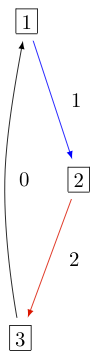In Sage this can be obtained via:

sage: K = crystals.KirillovReshetikhin(['A',2,1],1,1)
sage: G = K.digraph()
sage: view(G, tightpage=True) # optional - dot2tex graphviz, not tested (opens external window)


## Types $$D_n^{(1)}$$, $$B_n^{(1)}$$, $$A_{2n-1}^{(2)}$$¶

The Dynkin diagrams for types $$D_n^{(1)}$$, $$B_n^{(1)}$$, $$A_{2n-1}^{(2)}$$ are invariant under interchanging nodes $$0$$ and $$1$$:

sage: n = 5
sage: C = CartanType(['D',n,1]); C.dynkin_diagram()
0 O   O 5
|   |
|   |
O---O---O---O
1   2   3   4
D5~
sage: C = CartanType(['B',n,1]); C.dynkin_diagram()
O 0
|
|
O---O---O---O=>=O
1   2   3   4   5
B5~
sage: C = CartanType(['A',2*n-1,2]); C.dynkin_diagram()
O 0
|
|
O---O---O---O=<=O
1   2   3   4   5
B5~*


The underlying classical algebras obtained when removing node $$0$$ are type $$\mathfrak{g}_0 = D_n, B_n, C_n$$, respectively. The classical decomposition into a $$\mathfrak{g}_0$$ crystal is a direct sum:

$B^{r,s} \cong \bigoplus_\lambda B(\lambda) \quad \text{as a } \{1,2,\ldots,n\}\text{-crystal}$

where $$\lambda$$ is obtained from $$s\omega_r$$ (or equivalently a rectangular partition of shape $$(s^r)$$) by removing vertical dominoes. This in fact only holds in the ranges $$1\le r\le n-2$$ for type $$D_n^{(1)}$$, and $$1 \le r \le n$$ for types $$B_n^{(1)}$$ and $$A_{2n-1}^{(2)}$$:

sage: K = crystals.KirillovReshetikhin(['D',6,1],4,2)
sage: K.classical_decomposition()
The crystal of tableaux of type ['D', 6] and shape(s)
[[], [1, 1], [1, 1, 1, 1], [2, 2], [2, 2, 1, 1], [2, 2, 2, 2]]


For type $$B_n^{(1)}$$ and $$r=n$$, one needs to be aware that $$\omega_n$$ is a spin weight and hence corresponds in the partition language to a column of height $$n$$ and width $$1/2$$:

sage: K = crystals.KirillovReshetikhin(['B',3,1],3,1)
sage: K.classical_decomposition()
The crystal of tableaux of type ['B', 3] and shape(s) [[1/2, 1/2, 1/2]]


As for type $$A_n^{(1)}$$, the Dynkin automorphism induces a promotion-type operator $$\sigma$$ on the level of crystals. In this case in can however happen that the automorphism changes between classical components:

sage: K = crystals.KirillovReshetikhin(['D',4,1],2,1)
sage: b = K.module_generator(); b
[, ]
sage: K.automorphism(b)
[, [-1]]
sage: b = K(rows=[,[-2]])
sage: K.automorphism(b)
[]


This operator $$\sigma$$ is used to define the affine crystal operators:

\begin{align}\begin{aligned}f_0 = \sigma \circ f_1 \circ \sigma\\e_0 = \sigma \circ e_1 \circ \sigma\end{aligned}\end{align}

The KR crystals $$B^{1,1}$$ of types $$D_3^{(1)}$$, $$B_2^{(1)}$$, and $$A_5^{(2)}$$ are, respectively: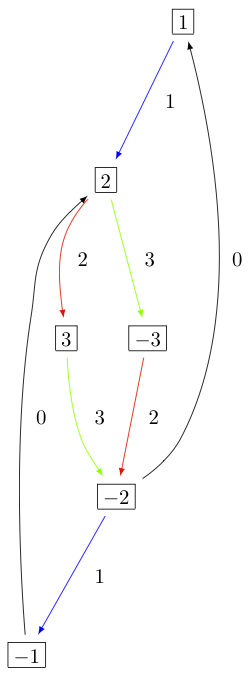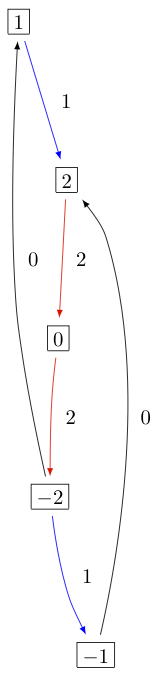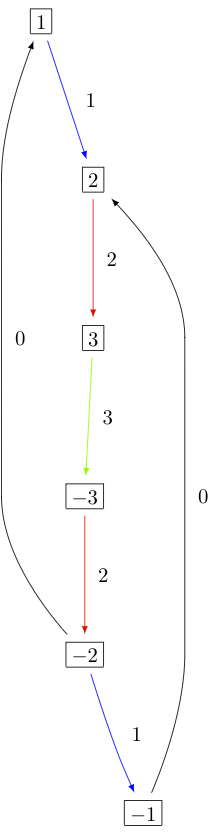## Type $$C_n^{(1)}$$¶

The Dynkin diagram of type $$C_n^{(1)}$$ has a symmetry $$\sigma(i) = n-i$$:

sage: C = CartanType(['C',4,1]); C.dynkin_diagram()
O=>=O---O---O=<=O
0   1   2   3   4
C4~


The classical subalgebra when removing the 0 node is of type $$C_n$$.

However, in this case the crystal $$B^{r,s}$$ is not constructed using $$\sigma$$, but rather using a virtual crystal construction. $$B^{r,s}$$ of type $$C_n^{(1)}$$ is realized inside $$\hat{V}^{r,s}$$ of type $$A_{2n+1}^{(2)}$$ using:

\begin{align}\begin{aligned}e_0 = \hat{e}_0 \hat{e}_1 \quad \text{and} \quad e_i = \hat{e}_{i+1} \quad \text{for} \quad 1\le i\le n\\f_0 = \hat{f}_0 \hat{f}_1 \quad \text{and} \quad f_i = \hat{f}_{i+1} \quad \text{for} \quad 1\le i\le n\end{aligned}\end{align}

where $$\hat{e}_i$$ and $$\hat{f}_i$$ are the crystal operator in the ambient crystal $$\hat{V}^{r,s}$$:

sage: K = crystals.KirillovReshetikhin(['C',3,1],1,2); K.ambient_crystal()
Kirillov-Reshetikhin crystal of type ['B', 4, 1]^* with (r,s)=(1,2)


The classical decomposition for $$1 \le r < n$$ is given by:

$B^{r,s} \cong \bigoplus_\lambda B(\lambda) \quad \text{as a } \{1,2,\ldots,n\}\text{-crystal}$

where $$\lambda$$ is obtained from $$s\omega_r$$ (or equivalently a rectangular partition of shape $$(s^r)$$) by removing horizontal dominoes:

sage: K = crystals.KirillovReshetikhin(['C',3,1],2,4)
sage: K.classical_decomposition()
The crystal of tableaux of type ['C', 3] and shape(s) [[], , , [2, 2], [4, 2], [4, 4]]


The KR crystal $$B^{1,1}$$ of type $$C_2^{(1)}$$ looks as follows: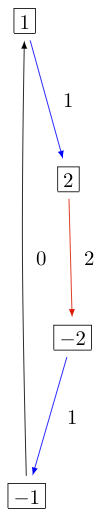## Types $$D_{n+1}^{(2)}$$, $$A_{2n}^{(2)}$$¶

The Dynkin diagrams of types $$D_{n+1}^{(2)}$$ and $$A_{2n}^{(2)}$$ look as follows:

sage: C = CartanType(['D',5,2]); C.dynkin_diagram()
O=<=O---O---O=>=O
0   1   2   3   4
C4~*

sage: C = CartanType(['A',8,2]); C.dynkin_diagram()
O=<=O---O---O=<=O
0   1   2   3   4
BC4~


The classical subdiagram is of type $$B_n$$ for type $$D_{n+1}^{(2)}$$ and of type $$C_n$$ for type $$A_{2n}^{(2)}$$. The classical decomposition for these KR crystals for $$1\le r < n$$ for type $$D_{n+1}^{(2)}$$ and $$1 \le r \le n$$ for type $$A_{2n}^{(2)}$$ is given by:

$B^{r,s} \cong \bigoplus_\lambda B(\lambda) \quad \text{as a } \{1,2,\ldots,n\}\text{-crystal}$

where $$\lambda$$ is obtained from $$s\omega_r$$ (or equivalently a rectangular partition of shape $$(s^r)$$) by removing single boxes:

sage: K = crystals.KirillovReshetikhin(['D',5,2],2,2)
sage: K.classical_decomposition()
The crystal of tableaux of type ['B', 4] and shape(s) [[], , , [1, 1], [2, 1], [2, 2]]

sage: K = crystals.KirillovReshetikhin(['A',8,2],2,2)
sage: K.classical_decomposition()
The crystal of tableaux of type ['C', 4] and shape(s) [[], , , [1, 1], [2, 1], [2, 2]]


The KR crystals are constructed using an injective map into a KR crystal of type $$C_n^{(1)}$$

$S : B^{r,s} \to B^{r,2s}_{C_n^{(1)}} \quad \text{such that } S(e_ib) = e_i^{m_i}S(b) \text{ and } S(f_ib) = f_i^{m_i}S(b)$

where

$(m_0,\ldots,m_n) = (1,2,\ldots,2,1) \text{ for type } D_{n+1}^{(2)} \quad \text{and} \quad (1,2,\ldots,2,2) \text{ for type } A_{2n}^{(2)}.$
sage: K = crystals.KirillovReshetikhin(['D',5,2],1,2); K.ambient_crystal()
Kirillov-Reshetikhin crystal of type ['C', 4, 1] with (r,s)=(1,4)
sage: K = crystals.KirillovReshetikhin(['A',8,2],1,2); K.ambient_crystal()
Kirillov-Reshetikhin crystal of type ['C', 4, 1] with (r,s)=(1,4)


The KR crystals $$B^{1,1}$$ of type $$D_3^{(2)}$$ and $$A_4^{(2)}$$ look as follows: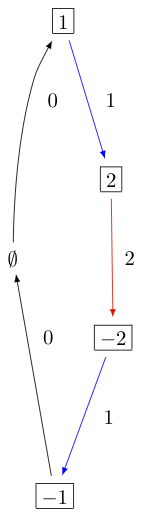As you can see from the Dynkin diagram for type $$A_{2n}^{(2)}$$, mapping the nodes $$i\mapsto n-i$$ yields the same diagram, but with relabelled nodes. In this case the classical subdiagram is of type $$B_n$$ instead of $$C_n$$. One can also construct the KR crystal $$B^{r,s}$$ of type $$A_{2n}^{(2)}$$ based on this classical decomposition. In this case the classical decomposition is the sum over all weights obtained from $$s \omega_r$$ by removing horizontal dominoes:

sage: C = CartanType(['A',6,2]).dual()
sage: Kdual = crystals.KirillovReshetikhin(C,2,2)
sage: Kdual.classical_decomposition()
The crystal of tableaux of type ['B', 3] and shape(s) [[], , [2, 2]]


Looking at the picture, one can see that this implementation is isomorphic to the other implementation based on the $$C_n$$ decomposition up to a relabeling of the arrows:

sage: C = CartanType(['A',4,2])
sage: K = crystals.KirillovReshetikhin(C,1,1)
sage: Kdual = crystals.KirillovReshetikhin(C.dual(),1,1)
sage: G = K.digraph()
sage: Gdual = Kdual.digraph()
sage: f = { 1:1, 0:2, 2:0 }
sage: for u,v,label in Gdual.edges():
....:     Gdual.set_edge_label(u,v,f[label])
sage: G.is_isomorphic(Gdual, edge_labels = True)
True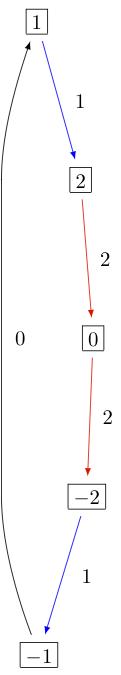## Exceptional nodes¶

The KR crystals $$B^{n,s}$$ for types $$C_n^{(1)}$$ and $$D_{n+1}^{(2)}$$ were excluded from the above discussion. They are associated to the exceptional node $$r=n$$ and in this case the classical decomposition is irreducible:

$B^{n,s} \cong B(s\omega_n).$

In Sage:

sage: K = crystals.KirillovReshetikhin(['C',2,1],2,1)
sage: K.classical_decomposition()
The crystal of tableaux of type ['C', 2] and shape(s) [[1, 1]]

sage: K = crystals.KirillovReshetikhin(['D',3,2],2,1)
sage: K.classical_decomposition()
The crystal of tableaux of type ['B', 2] and shape(s) [[1/2, 1/2]]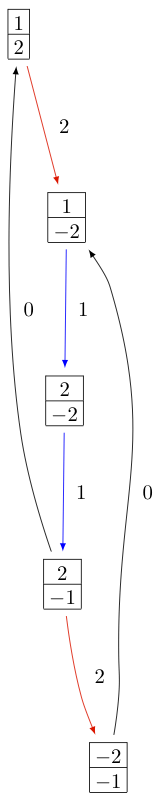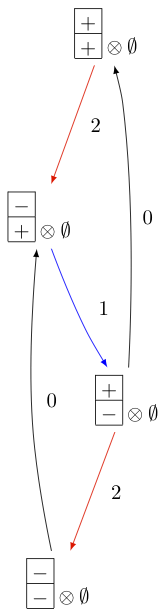The KR crystals $$B^{n,s}$$ and $$B^{n-1,s}$$ of type $$D_n^{(1)}$$ are also special. They decompose as:

$B^{n,s} \cong B(s\omega_n) \quad \text{ and } \quad B^{n-1,s} \cong B(s\omega_{n-1}).$
sage: K = crystals.KirillovReshetikhin(['D',4,1],4,1)
sage: K.classical_decomposition()
The crystal of tableaux of type ['D', 4] and shape(s) [[1/2, 1/2, 1/2, 1/2]]
sage: K = crystals.KirillovReshetikhin(['D',4,1],3,1)
sage: K.classical_decomposition()
The crystal of tableaux of type ['D', 4] and shape(s) [[1/2, 1/2, 1/2, -1/2]]


## Type $$E_6^{(1)}$$¶

In [JonesEtAl2010] the KR crystals $$B^{r,s}$$ for $$r=1,2,6$$ in type $$E_6^{(1)}$$ were constructed exploiting again a Dynkin diagram automorphism, namely the automorphism $$\sigma$$ of order 3 which maps $$0\mapsto 1 \mapsto 6 \mapsto 0$$:

sage: C = CartanType(['E',6,1]); C.dynkin_diagram()
O 0
|
|
O 2
|
|
O---O---O---O---O
1   3   4   5   6
E6~


The crystals $$B^{1,s}$$ and $$B^{6,s}$$ are irreducible as classical crystals:

sage: K = crystals.KirillovReshetikhin(['E',6,1],1,1)
sage: K.classical_decomposition()
Direct sum of the crystals Family (Finite dimensional highest weight crystal of type ['E', 6] and highest weight Lambda,)
sage: K = crystals.KirillovReshetikhin(['E',6,1],6,1)
sage: K.classical_decomposition()
Direct sum of the crystals Family (Finite dimensional highest weight crystal of type ['E', 6] and highest weight Lambda,)


whereas for the adjoint node $$r=2$$ we have the decomposition

$B^{2,s} \cong \bigoplus_{k=0}^s B(k\omega_2)$
sage: K = crystals.KirillovReshetikhin(['E',6,1],2,1)
sage: K.classical_decomposition()
Direct sum of the crystals Family (Finite dimensional highest weight crystal of type ['E', 6] and highest weight 0,
Finite dimensional highest weight crystal of type ['E', 6] and highest weight Lambda)


The promotion operator on the crystal corresponding to $$\sigma$$ can be calculated explicitly:

sage: K = crystals.KirillovReshetikhin(['E',6,1],1,1)
sage: promotion = K.promotion()
sage: u = K.module_generator(); u
[(1,)]
sage: promotion(u.lift())
[(-1, 6)]


The crystal $$B^{1,1}$$ is already of dimension 27. The elements $$b$$ of this crystal are labelled by tuples which specify their nonzero $$\phi_i(b)$$ and $$\epsilon_i(b)$$. For example, $$[-6,2]$$ indicates that $$\phi_2([-6,2]) = \epsilon_6([-6,2]) = 1$$ and all others are equal to zero:

sage: K = crystals.KirillovReshetikhin(['E',6,1],1,1)
sage: K.cardinality()
27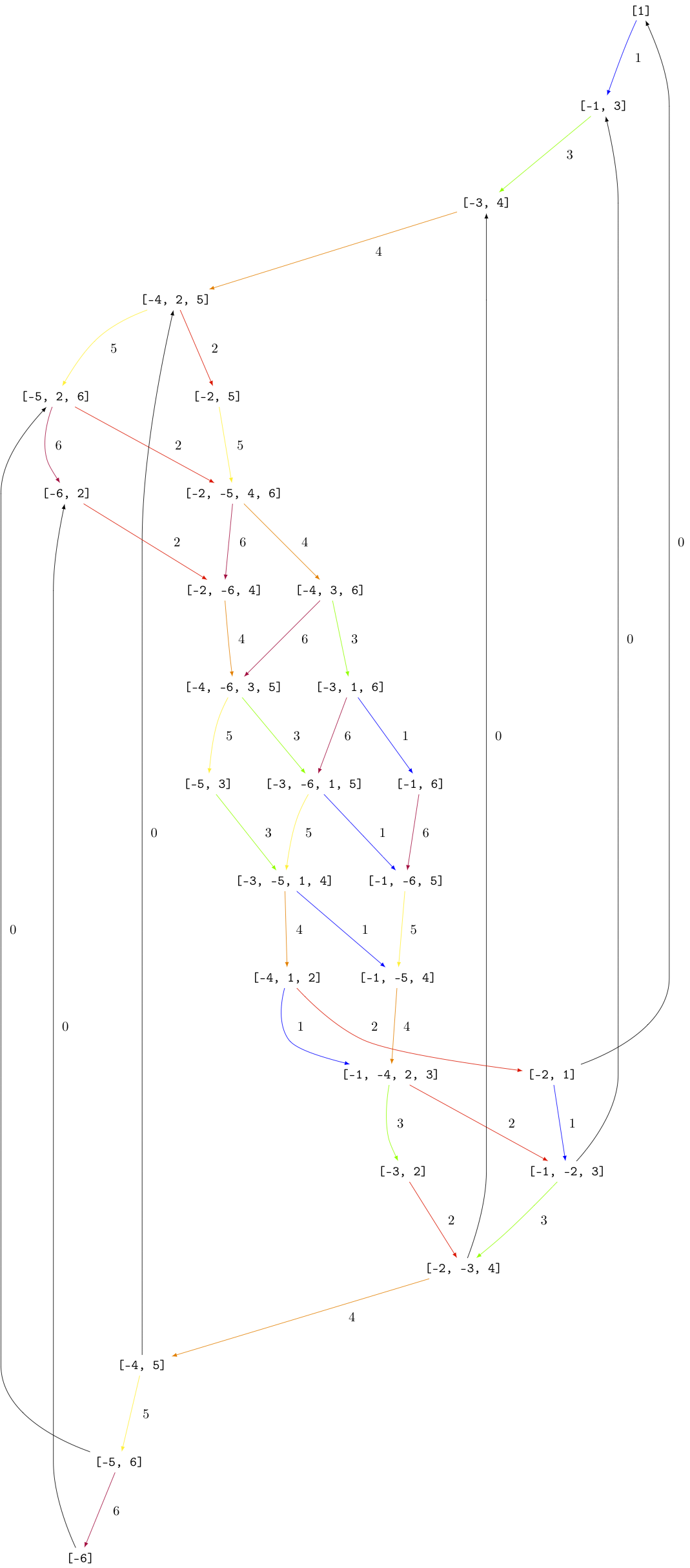## Single column KR crystals¶

A single column KR crystal is $$B^{r,1}$$ for any $$r \in I_0$$.

In [LNSSS14I] and [LNSSS14II], it was shown that single column KR crystals can be constructed by projecting level 0 crystals of LS paths onto the classical weight lattice. We first verify that we do get an isomorphic crystal for $$B^{1,1}$$ in type $$E_6^{(1)}$$:

sage: K = crystals.KirillovReshetikhin(['E',6,1], 1,1)
sage: K2 = crystals.kirillov_reshetikhin.LSPaths(['E',6,1], 1,1)
sage: K.digraph().is_isomorphic(K2.digraph(), edge_labels=True)
True


Here is an example in $$E_8^{(1)}$$ and we calculate its classical decomposition:

sage: K = crystals.kirillov_reshetikhin.LSPaths(['E',8,1], 8,1)
sage: K.cardinality()
249
sage: L = [x for x in K if x.is_highest_weight([1,2,3,4,5,6,7,8])]
sage: [x.weight() for x in L]
[-2*Lambda + Lambda, 0]


## Applications¶

An important notion for finite-dimensional affine crystals is perfectness. The crucial property is that a crystal $$B$$ is perfect of level $$\ell$$ if there is a bijection between level $$\ell$$ dominant weights and elements in

$B_{\mathrm{min}} = \{ b \in B \mid \mathrm{lev}(\varphi(b)) = \ell \}\;.$

For a precise definition of perfect crystals see [HongKang2002] . In [FourierEtAl2010] it was proven that for the nonexceptional types $$B^{r,s}$$ is perfect as long as $$s/c_r$$ is an integer. Here $$c_r=1$$ except $$c_r=2$$ for $$1 \le r < n$$ in type $$C_n^{(1)}$$ and $$r=n$$ in type $$B_n^{(1)}$$.

Here we verify this using Sage for $$B^{1,1}$$ of type $$C_3^{(1)}$$:

sage: K = crystals.KirillovReshetikhin(['C',3,1],1,1)
sage: Lambda = K.weight_lattice_realization().fundamental_weights(); Lambda
Finite family {0: Lambda, 1: Lambda, 2: Lambda, 3: Lambda}
sage: [w.level() for w in Lambda]
[1, 1, 1, 1]
sage: Bmin = [b for b in K if b.Phi().level() == 1 ]; Bmin
[[], [], [], [[-3]], [[-2]], [[-1]]]
sage: [b.Phi() for b in Bmin]
[Lambda, Lambda, Lambda, Lambda, Lambda, Lambda]


As you can see, both $$b=1$$ and $$b=-2$$ satisfy $$\varphi(b)=\Lambda_1$$. Hence there is no bijection between the minimal elements in $$B_{\mathrm{min}}$$ and level 1 weights. Therefore, $$B^{1,1}$$ of type $$C_3^{(1)}$$ is not perfect. However, $$B^{1,2}$$ of type $$C_n^{(1)}$$ is a perfect crystal:

sage: K = crystals.KirillovReshetikhin(['C',3,1],1,2)
sage: Lambda = K.weight_lattice_realization().fundamental_weights()
sage: Bmin = [b for b in K if b.Phi().level() == 1 ]
sage: [b.Phi() for b in Bmin]
[Lambda, Lambda, Lambda, Lambda]


Perfect crystals can be used to construct infinite-dimensional highest weight crystals and Demazure crystals using the Kyoto path model [KKMMNN1992]. We construct Example 10.6.5 in [HongKang2002]:

sage: K = crystals.KirillovReshetikhin(['A',1,1], 1,1)
sage: La = RootSystem(['A',1,1]).weight_lattice().fundamental_weights()
sage: B = crystals.KyotoPathModel(K, La)
sage: B.highest_weight_vector()
[[]]

sage: K = crystals.KirillovReshetikhin(['A',2,1], 1,1)
sage: La = RootSystem(['A',2,1]).weight_lattice().fundamental_weights()
sage: B = crystals.KyotoPathModel(K, La)
sage: B.highest_weight_vector()
[[]]

sage: K = crystals.KirillovReshetikhin(['C',2,1], 2,1)
sage: La = RootSystem(['C',2,1]).weight_lattice().fundamental_weights()
sage: B = crystals.KyotoPathModel(K, La)
sage: B.highest_weight_vector()
[[, [-2]]]


## Energy function and one-dimensional configuration sum¶

For tensor products of Kirillov-Reshehtikhin crystals, there also exists the important notion of the energy function. It can be defined as the sum of certain local energy functions and the $$R$$-matrix. In Theorem 7.5 in [SchillingTingley2011] it was shown that for perfect crystals of the same level the energy $$D(b)$$ is the same as the affine grading (up to a normalization). The affine grading is defined as the minimal number of applications of $$e_0$$ to $$b$$ to reach a ground state path. Computationally, this algorithm is a lot more efficient than the computation involving the $$R$$-matrix and has been implemented in Sage:

sage: K = crystals.KirillovReshetikhin(['A',2,1],1,1)
sage: T = crystals.TensorProduct(K,K,K)
sage: hw = [b for b in T if all(b.epsilon(i)==0 for i in [1,2])]
sage: for b in hw:
....:     print("{} {}".format(b, b.energy_function()))
[[], [], []] 0
[[], [], []] 2
[[], [], []] 1
[[], [], []] 3


The affine grading can be computed even for nonperfect crystals:

sage: K = crystals.KirillovReshetikhin(['C',4,1],1,2)
sage: K1 = crystals.KirillovReshetikhin(['C',4,1],1,1)
sage: T = crystals.TensorProduct(K,K1)
sage: hw = [b for b in T if all(b.epsilon(i)==0 for i in [1,2,3,4])]
sage: for b in hw:
[[], []] 1
[[[1, 1]], []] 2
[[[1, 2]], []] 1
[[[1, -1]], []] 0


The one-dimensional configuration sum of a crystal $$B$$ is the graded sum by energy of the weight of all elements $$b \in B$$:

$X(B) = \sum_{b \in B} x^{\mathrm{weight}(b)} q^{D(b)}$

Here is an example of how you can compute the one-dimensional configuration sum in Sage:

sage: K = crystals.KirillovReshetikhin(['A',2,1],1,1)
sage: T = crystals.TensorProduct(K,K)
sage: T.one_dimensional_configuration_sum()
B[-2*Lambda + 2*Lambda] + (q+1)*B[-Lambda]
+ (q+1)*B[Lambda - Lambda] + B[2*Lambda]
+ B[-2*Lambda] + (q+1)*B[Lambda]